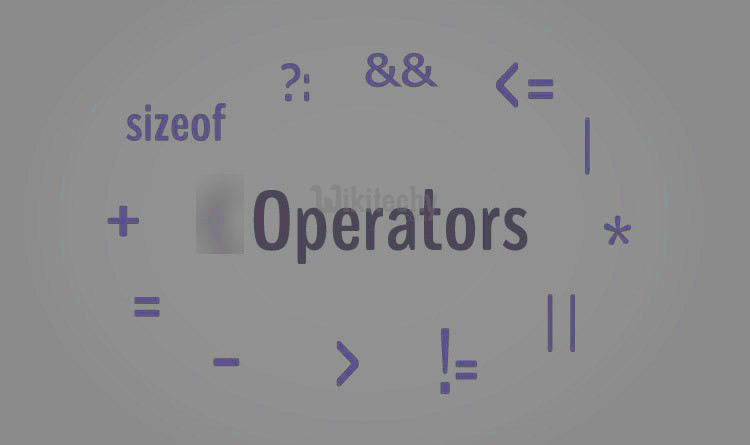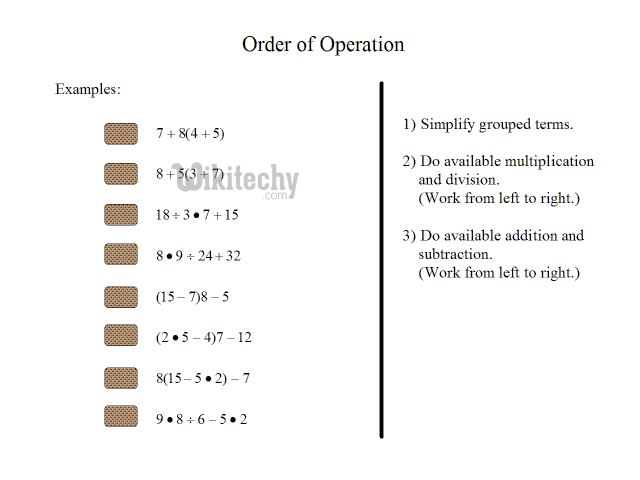# Operator C++ | Operators and its operations - Learn C++ , C++ Tutorial , C++ programming

## What is C++ Operator?

• An operator is a symbol that expresses the compiler to perform specific mathematical or logical manipulations.
•learn c++ tutorials - operators Example

• C++ is rich in built-in operators and provides the following types of operators:
• Arithmetic Operators
• Relational Operators
• Logical Operators
• Bitwise Operators
• Assignment Operators
• Misc Operators
• This chapter will show the arithmetic, relational, logical, bitwise, assignment and other operators one by one## Arithmetic Operators

• It provides operators for five basic arithmetic calculations: addition, subtraction, multiplication, division, and taking the modulus.
• Each of these operators uses two values (called operands) to calculate a final answer.
•learn c++ tutorials - operators-operands Example

• There are following arithmetic operators supported by C++ language:

Assume variable A holds 10 and variable B holds 20, then:

## Show Examples

Operator Description Example
+ Adds two operands A + B will give 30
- Subtracts second operand from the first A - B will give -10
* Multiplies both operandstd A * B will give 200
/ Divides numerator by de-numerator B / A will give 2
% Modulus Operator and remainder of after an integer division B % A will give 0
++ Increment operator, increases integer value by one A++ will give 11
-- Decrement operator, decreases integer value by one A-- will give 9

## Relational Operators

• A relational operator is a programming language construct oroperator that tests or defines some kind of relation between two entities.
• These include numerical equality (e.g., 5 = 5) and inequalities (e.g., 4 ≥ 3)
• There are following relational operators supported by C++ language

Assume variable A holds 10 and variable B holds 20, then:

## Show Examples

Operator Description Example
== Checks if the values of two operands
are equal or not, if yes then condition becomes true.
(A == B) is not true.
!= Checks if the values of two operands are equal or
not, if values are not equal then condition becomes true.
(A != B) is true.
> Checks if the value of left operand is greater than the
value of right operand, if yes then condition becomes true.
(A > B) is not true.
< Checks if the value of left operand is less than the
value of right operand, if yes then condition becomes true.
(A < B) is true.
>= Checks if the value of left operand is greater than or
equal to the value of right operand, if yes then condition becomes true.
(A >= B) is not true.
<= Checks if the value of left operand is less than or equal to the value
of right operand, if yes then condition becomes true.
(A <= B) is true.

## Logical Operators

• Logical Operators are used if we want to compare more than one condition.
• Depending upon the requirement, proper logical operator is used.
• There are following logical operators supported by C++ language

Assume variable A holds 1 and variable B holds 0, then:

## Show Examples

Operator Description Example
&& Called Logical AND operator. If both the operands are non-zero, then condition becomes true. (A && B) is false.
>|| Called Logical OR Operator. If any of the two operands is non-zero, then condition becomes true. (A || B) is true.
! Called Logical NOT Operator. Use to reverses the
logical state of its operand. If a condition is true, then Logical NOT operator will make false.
!(A && B) is true.

## Bitwise Operators

• Bitwise operator works on bits and perform bit-by-bit operation.
• The truth tables for &, |, and ^ are as follows:
p q p & q p | q p ^ q
0 0 0 0 0
0 1 0 1 0
1 1 1 1 0
1 0 0 1 1

Assume if A = 60; and B = 13; now in binary format they will be as follows:

• The Bitwise operators supported by C++ language are listed in the following table. Assume variable A holds 60 and variable B holds 13, then:

## Show Examples

OperatorDescriptionExample
& Binary AND Operator copies a bit to the
result if it exists in both operands.
(A & B) will give 12 which is 0000 1100
| Binary OR Operator copies a bit if it
exists in either operand.
(A | B) will give 61 which is 0011 1101
^ Binary XOR Operator copies the bit
if it is set in one operand but not both.
(A ^ B) will give
49 which is 0011 0001
~ Binary Ones Complement Operator is
unary and has the effect of 'flipping' bits.
(~A ) will give -61 which is 1100 0011 in 2's complement form
due to a signed binary number.
<< Binary Left Shift Operator. The left operands
value is moved left by the
number of bits specified by the left operand.
A << 2 will give 240 which is 1111 0000
>> Binary Right Shift Operator.
The left operands value is moved
right by the number of bits specified by the right operand.
A >> 2 will give 15 which is 0000 1111

## Assignment Operators

• An assignment statement sets and/or re-sets the value stored in the storage location(s) denoted by a variable name.
• In other words, it copies a value into the variable
• There are following assignment operators supported by C++ language:

## Show Examples

OperatorDescriptionExample
=Simple assignment operator, Assigns values
from right side operands to left side operand
C = A + B will assign value of A + B into C
operand to the left operand and assign the result to left operand
C += A is equivalent to C = C + A
-=Subtract AND assignment operator, It subtracts right
operand from the left operand and assign the result to left operand
C -= A is equivalent to C = C - A
*=Multiply AND assignment operator, It multiplies right
operand with the left operand and assign the result to left operand
C *= A is equivalent to C = C * A
/=Divide AND assignment operator, It divides left operand with the
right operand and assign the result to left operand
C /= A is equivalent to C = C / A
%=Modulus AND assignment operator, It takes modulus using two
operands and assign the result to left operand
C %= A is equivalent to C = C % A
<<=Left shift AND assignment operator C <<= 2 is same as C = C << 2
>>=Right shift AND assignment operator C >>= 2 is same as C = C >> 2
&=Bitwise AND assignment operator C &= 2 is same as C = C & 2
^=bitwise exclusive OR and assignment operator C ^= 2 is same as C = C ^ 2
|=bitwise inclusive OR and assignment operator C |= 2 is same as C = C | 2

## Misc Operators

• There are few other operators supported by C++ Language.
OperatorDescription
sizeofsizeof operator returns the size of a variable. For example, sizeof(a), where a is integer, will return 4.
Condition ? X : YConditional operator. If Condition is true ? then it returns value X : otherwise value Y
,Comma operator causes a sequence of operations to be performed. The value of the entire comma expression is the value of the last expression of the comma-separated list.
. (dot) and -> (arrow)Member operators are used to reference individual members of classes, structures, and unions.
CastCasting operators convert one data type to another. For example, int(2.2000) would return 2.
&Pointer operator & returns the address of an variable. For example &a; will give actual address of the variable.
*Pointer operator * is pointer to a variable. For example *var; will pointer to a variable var.

## Operators Precedence in C++

• Operator precedence determines the grouping of terms in an expression.
• This affects how an expression is evaluated. Certain operators have higher precedence than others;
•learn c++ tutorials - operator precedence in c++ Example

• for example, the multiplication operator has higher precedence than the addition operator:
• For example x = 7 + 3 * 2; here, x is assigned 13, not 20 because operator * has higher precedence than +, so it first gets multiplied with 3*2 and then adds into 7.
• Here, operators with the highest precedence appear at the top of the table, those with the lowest appear at the bottom. Within an expression, higher precedence operators will be evaluated first.

## Show Examples

Category  Operator Associativity
Postfix () [] -> . ++ - -   Left to right
Unary  + - ! ~ ++ - - (type)* & sizeof  Right to left
Multiplicative   * / % Left to right
Additive  + -  Left to right
Shift   << >>  Left to right
Relational  < <= > >=  Left to right
Equality   == !=  Left to right
Bitwise AND  Left to right
Bitwise XOR  Left to right
Bitwise OR  Left to right
Logical AND &&  Left to right
Logical OR  ||  Left to right
Conditional ?:  Right to left
Assignment  = += -= *= /= %=>>= <<= &= ^= |= Right to left
Comma  Left to right